## Cylinder Function

The cylinder function is defined as(1)

The Bessel Functions are sometimes also called cylinder functions. To find the Fourier Transform of the cylinder function, let(2)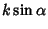(3)(4)(5)

Then(6)

Let, so. Then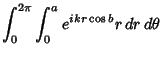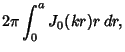(7)

whereis a zeroth order Bessel Function of the First Kind. Let, so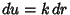, then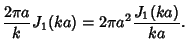(8)

As defined by Watson (1966), a cylinder function'' is any function which satisfies the Recurrence Relations(9)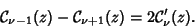(10)

This class of functions can be expressed in terms of Bessel Functions.

See also Bessel Function of the First Kind, Cylinder Function, Cylindrical Function, Hemispherical Function

References

Watson, G. N. A Treatise on the Theory of Bessel Functions, 2nd ed. Cambridge, England: Cambridge University Press, 1966.